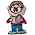Friday, March 20, 2009

Finish the Square

Logically, what number should replace the ?

6 8 12
10 2 5
15 4 ?

1.30?

2.15 is the answer.

Going either from top to bottom or from left two right along any column or any row, respectively, the third number is the product of the first and second numbers divided by four.

For example, the rows are:
6 * 8 = 48 / 4 = 12
10 * 2 = 20 / 4 = 5
15 * 4 = 60 / 4 = 15

and the columns are:
6 * 10 = 60 / 4 = 15
8 * 2 = 16 / 4 = 4
12 * 5 = 60 / 4 = 15.

3.15 is the answer. As oudeis explained, the rows and columns all work out to x*y/4.

Leave your answer or, if you want to post a question of your own, send me an e-mail. Look in the about section to find my e-mail address. If it's new, I'll post it soon.

Please don't leave spam or 'Awesome blog, come visit mine' messages. I'll delete them soon after.

Enter your Email and join hundreds of others who get their Question of the Day sent right to their mailbox

The Lamplight Manor Puzz 3-D